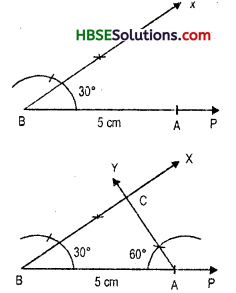# HBSE 7th Class Maths Solutions Chapter 10 Practical Geometry Ex 10.4

Haryana State Board HBSE 7th Class Maths Solutions Chapter 10 Practical Geometry Ex 10.4 Textbook Exercise Questions and Answers.

## Haryana Board 7th Class Maths Solutions Chapter 10 Practical Geometry  Exercise 10.4

Question 1.
Construct ΔABC, given m∠A = 60°, m∠B = 30° and AB = 5 cm.
Solution:
Draw a rough sketch of ΔABC and indicate the measures of the two angles and length of the included side.
Steps of Construction :
1. Draw a ray BP.
2. From ray BP, cut off a line-segment BA = 5 cm.3. At M, construct ∠XBA of measure 30°. (Fig.)
4. At A construct ∠YAB of measure 60°. Let the rays BX and AY intersect at C. Then ΔABC is the required triangle. (Fig.)Question 2.
Construct ΔPQR if PQ = 5 cm, m∠PQR = 105° and m∠QRP = 40°. (Hint : Recall angle-sum property of a triangle).
Solution:
Angle sum property of a triangle
∠P + ∠Q + ∠R = 180°
∠P + 105° + 40° = 180°
or, ∠P = 180°-145°
∴ ∠P = 35°
Steps of Construction :
1. Construct PQ = 5 cm.
2. Using protractor, draw ∠Q = 105° and ∠P = 35°.
3. Let their new arms meet at R. Now ΔPQR is the required triangle.Question 3.
Examine whether you can construct ΔDEF such that EF = 7.2 cm, m∠E = 110° and m∠F = 80°. Justify your answer.
Solution:
In ΔDEF, EF = 7.2 cm and
∠E + ∠F + ∠D = 180°
But, 110+ 80 +∠D ≠ 180°
or, 190° + ∠D ≠ 180°
Which is not possible as the sum of three angles of a triangle is 180°, So this triangle cannot be constructed.